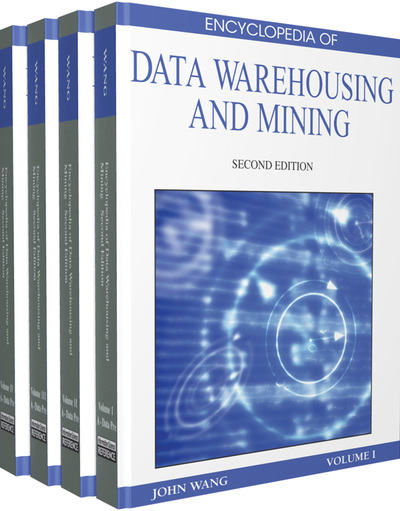# Formal Concept Analysis Based Clustering

Jamil M. Saquer (Southwest Missouri State University, USA)
DOI: 10.4018/978-1-60566-010-3.ch138

## Abstract

Formal concept analysis (FCA) is a branch of applied mathematics with roots in lattice theory (Wille, 1982; Ganter & Wille, 1999). It deals with the notion of a concept in a given universe, which it calls context. For example, consider the context of transactions at a grocery store where each transaction consists of the items bought together. A concept here is a pair of two sets (A, B). A is the set of transactions that contain all the items in B and B is the set of items common to all the transactions in A. A successful area of application for FCA has been data mining. In particular, techniques from FCA have been successfully used in the association mining problem and in clustering (Kryszkiewicz, 1998; Saquer, 2003; Zaki & Hsiao, 2002). In this article, we review the basic notions of FCA and show how they can be used in clustering.
Chapter Preview
Top

## Background

A fundamental notion in FCA is that of a context, which is defined as a triple (G, M, I), where G is a set of objects, M is a set of features (or attributes), and I is a binary relation between G and M. For object g and feature m, gIm if and only if g possesses the feature m. An example of a context is given in Table 1, where an “X” is placed in the ith row and jth column to indicate that the object in row i possesses the feature in column j.

Table 1.
A context excerpted from (Ganter, and Wille, 1999, p. 18). a = needs water to live; b = lives in water; c = lives on land; d = needs chlorophyll; e = two seeds leaf; f = one seed leaf; g = can move around; h = has limbs; i = suckles its offsprings.
 a b c d e f g h i 1 Leech X X X 2 Bream X X X X 3 Frog X X X X X 4 Dog X X X X X 5 Spike-weed X X X X 6 Reed X X X X X 7 Bean X X X X 8 Maize X X X X

## Complete Chapter List

Search this Book:
Reset